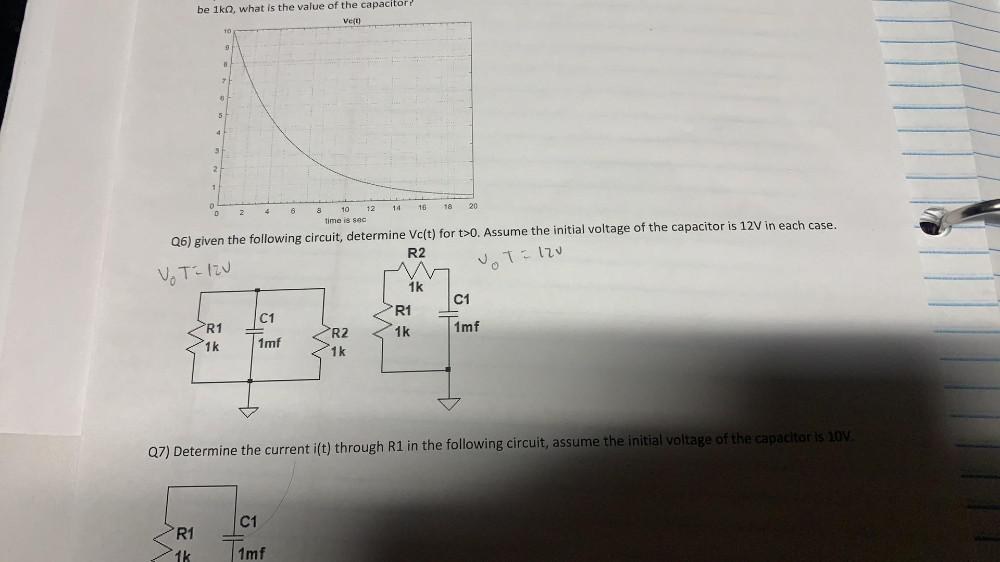Question:

# be 1kn2, what is the value of the capacitor? Ve(t) 10 9 8 7 6 5 4 3 2. 1 0 0 16 14 20 18 6 2 4 10 12 time is sec Q6) given the fbe 1kn2, what is the value of the capacitor? Ve(t) 10 9 8 7 6 5 4 3 2. 1 0 0 16 14 20 18 6 2 4 10 12 time is sec Q6) given the following circuit, determine Vc(t) for t>O. Assume the initial voltage of the capacitor is 12V in each case. R2 vot=120 Vo Talv C1 C1 R1 1k R1 R2 1mf imf 1k 1k Q7) Determine the current i(t) through R1 in the following circuit, assume the initial voltage of the capacitor is 10V. C1 R1 1mf